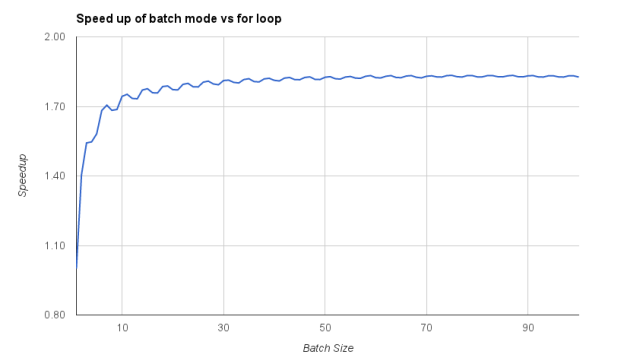# ArrayFire: A Portable Open-Source Accelerated Computing LibraryThe ArrayFire library is a high-performance software library with a focus on portability and productivity. It supports highly tuned, GPU-accelerated algorithms using an easy-to-use API. ArrayFire wraps GPU memory into a simple “array” object, enabling developers to process vectors, matrices, and volumes on the GPU using high-level routines, without having to get involved with device kernel code.

## ArrayFire Capabilities

ArrayFire is an open-source C/C++ library, with language bindings for R, Java and Fortran. ArrayFire has a range of functionality, including

ArrayFire has three back ends to enable portability across many platforms: CUDA, OpenCL and CPU. It even works on embedded platforms like NVIDIA’s Jetson TK1.

In a past post about ArrayFire we demonstrated the ArrayFire capabilities and how you can increase your productivity by using ArrayFire. In this post I will tell you how you can use ArrayFire to exploit various kind of parallelism on NVIDIA GPUs.

## Batch Operations

The ability to work with multiple datasets in parallel is both necessary and important in many applications. ArrayFire facilitates such operations by enabling batch support for all functions. ArrayFire infers the batch size from the additional dimensions passed to a function. For example, image processing functions treat the third dimension of the inputs as the batch size (number of images).

Here is an example demonstrating the batch capabilities of ArrayFire.

```static void bench_rotate(int N)
{
for (int num = 1; num <= N; num++) {
// Generate "num" random images of size 1920 x 1080
array imgs = randu(1920, 1080, num);
af::sync();
timer::start();

for (int i = 0; i < iters; i++) {
// Rotate an image by 90 degrees
array rot = rotate(imgs, PI / 2);
}

af::sync();
double t = 1000 * timer::stop();

printf("Average time taken for %2d imags: %4.4lfmsn", num, t/iters/num);
}
}
```

### Benchmark Results

I generated the following graph using the times from the code shown above on an NVIDIA Tesla K40 accelerator. You can see that using batch mode in ArrayFire gradually increases the overall throughput before saturating at an 80% speedup (compared to iteratively performing all the rotations).Speedup of GPU-accelerated 1920×1080 image rotation in ArrayFire with increasing batch size (number of images rotated).

## Parallelism Along Any Dimension

Manycore algorithms in ArrayFire can perform an operation along any of the dimensions of the input. Many simulation algorithms perform numerical differentiation along various dimensions on the input data. ArrayFire makes this easy, as the following code snippet shows.

```static void diff_demo()
{
array in = randu(2, 2, 2);

array dx = diff1(in, 0); // Numerical difference along x
array dy = diff1(in, 1); // Numerical difference along y
array dz = diff1(in, 2); // Numerical difference along z

af_print(in);
af_print(dx);
af_print(dy);
af_print(dz);
}
```

Output:

```in [2 2 2 1]
0.7402 0.0390
0.9210 0.9690

0.9251 0.6673
0.4464 0.1099

dx [1 2 2 1]
0.1808 0.9299

-0.4788 -0.5574

dy [2 1 2 1]
-0.7012
0.0480

-0.2578
-0.3364

dz [2 2 1 1]
0.1849 0.6283
-0.4746 -0.8590
```

## Nested Batch Operations

Some applications have two batched inputs, requiring an all-to-all operation. A couple of common examples include k-nearest neighbors (distance matrix) and Convolutional Neural Networks (convolution on multiple inputs, multiple filters).

ArrayFire provides data manipulation routines that make it easier for users to convert data into more parallelizable formats. In this example we show how you can calculate a distance matrix in parallel.

```static array dist_tile2(array a, array b)
{
int feat_len = a.dims(0);
int alen = a.dims(1);
int blen = b.dims(1);

// Shape of a is (feat_len, alen, 1)
array a_mod = a;
// Reshape b from (feat_len, blen) to (feat_len, 1, blen)
array b_mod = moddims(b, feat_len, 1, blen);

// Tile both matrices to be (feat_len, alen, blen)
array a_tiled = tile(a_mod, 1, 1, blen);
array b_tiled = tile(b_mod, 1, alen, 1);

// Do The sum operation along first dimension
// Output is of shape (1, alen, blen)
array dist_mod = sum(abs(a_tiled - b_tiled));

// Reshape dist_mat from (1, alen, blen) to (alen, blen)
array dist_mat = moddims(dist_mod, alen, blen);
return dist_mat;
}
```

You can read more about vectorization techniques in ArrayFire here. These techniques, while requiring significant GPU memory for large matrices, scale easily across multiple GPUs.

## Just-In-Time Kernel Generation

To reduce the overhead of launching multiple kernels and global memory accesses, ArrayFire groups element-wise operations using a Just-In-Time (JIT) framework. This reduces the total number of kernels launched and the number of temporary buffers required.

The following example demonstrates Monte Carlo estimation of Pi using ArrayFire.

```static double calc_pi(const int samples)
{
// Generate millions of random points on a plane
array x = randu(samples,f32), y = randu(samples,f32);
// Calculate how many points fall within a unit circle
return 4.0 * sum(sqrt(x*x + y*y) < 1) / samples;
}
```

Without JIT, this function would require eight kernel launches. Two for randu, two for *, one for +, one for sqrt, one for <, and one for sum. The JIT merges the element-wise operations into a single kernel using NVVM IR (NVIDIA’s LLVM-based compiler Intermediate Representation). Here is part of the generated LLVM code.

```core:

%inIdx0 = getelementptr inbounds float* %in0, i64 %idx

%inIdx1 = getelementptr inbounds float* %in1, i64 %idx

%val2 = call float @___mulss(float %val0, float %val1)
%inIdx3 = getelementptr inbounds float* %in3, i64 %idx

%inIdx4 = getelementptr inbounds float* %in4, i64 %idx

%val5 = call float @___mulss(float %val3, float %val4)
%val6 = call float @___addss(float %val2, float %val5)
%val7 = call float @___sqrts(float %val6)
%val9 = call i8 @___ltss(float %val7, float %val8)
%outIdx = getelementptr inbounds i8* %out, i64 %idx
store i8 %val9, i8* %outIdx

ret void
```

## Getting Started with ArrayFire

As I mentioned, ArrayFire is now open-source. If you want to get started with ArrayFire, read the wiki and our getting started page.

You can also learn more about ArrayFire from the upcoming GTC Express Webinar this Wednesday, December 10, 2014 at 9am PST.